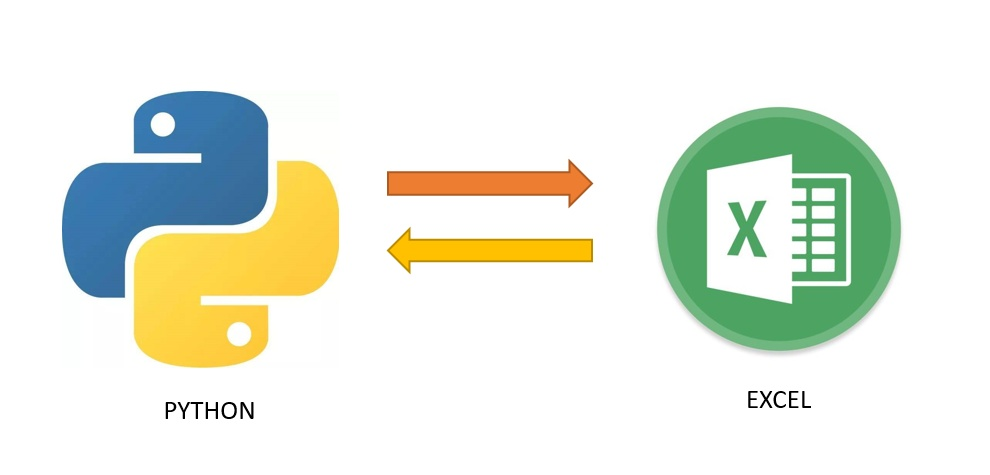• pandas to_excel:写入数据，写入到多个sheet里，在同一个sheet中追加数据 一、写入数据 了解下to_excel 的其他参数，写入操作非常简单 df.to_excel('aa.xlsx') 二、写入到多个sheet中 这个就和之前写过的“解决...
pandas to_excel:写入数据，写入到多个sheet里，在同一个sheet中追加数据
一、写入数据
了解下to_excel 的其他参数，写入操作非常简单
df.to_excel('aa.xlsx')

二、写入到多个sheet中
这个就和之前写过的“解决pandas中to_excel 数据覆盖sheet表问题”是差不多的，如果要实现同时写多个sheet的话，加一个循环或者判断就好。现在下面给“解决pandas中to_excel 数据覆盖sheet表问题”的代码。可以针对sheet_name做修改或者加一个循环
import pandas as pd
from openpyxl import load_workbook
result2=[('a','2','ss'),('b','2','33'),('c','4','bbb')]#列表数据
writer = pd.ExcelWriter('123.xlsx',engine='openpyxl')#可以向不同的sheet写入数据
book=load_workbook('123.xlsx')
writer.book = book
df = pd.DataFrame(result2,columns=['xuhao','id','name'])#列表数据转为数据框
df.to_excel(writer, sheet_name='sheet2')#将数据写入excel中的sheet2表,sheet_name改变后即是新增一个sheet
writer.save()#保存


三、在同一个sheet中追加数据
pd.to_csv()的话就非常方便，直接使参数mode='a’即可，但是to_excel没有这个参数，所以只能从侧面来实现了。这里主要就是限制开始写入的行（startrow）。
逻辑是：通过数据框获取到该Excel表的行数 df_rows，然后将需要存储的数据，限制开始写入的行数，即：startrow=df_rows+1 （原表中第一个空行），然后写入到同一个sheet中。这个方法不会覆盖原来的数据，实现在同一个sheet中追加数据。
代码如下：
注意，写入时需设置不包含列序号，行序号，即header=False，index=False
import pandas as pd
from openpyxl import load_workbook
result2=[('a','2','ss'),('b','2','33'),('c','4','bbb')]#需要新写入的数据
df = pd.DataFrame(result2,columns=['xuhao','id','name'])#列表数据转为数据框
df1 = pd.DataFrame(pd.read_excel('123.xlsx',sheet_name='aa')) #读取原数据文件和表
writer = pd.ExcelWriter('123.xlsx',engine='openpyxl')
book=load_workbook('123.xlsx')
writer.book = book
writer.sheets = dict((ws.title, ws) for ws in book.worksheets)
df_rows = df1.shape #获取原数据的行数
df.to_excel(writer, sheet_name='aa',startrow=df_rows+1, index=False, header=False)#将数据写入excel中的aa表,从第一个空行开始写
writer.save()#保存



展开全文python 经验分享
• 使用pandas 的to_excel()函数往一个excel文件写入多个sheet页时要使用如下方法： writer = pd.ExcelWriter(filepath) df1.to_excel(excel_writer=writer,sheet_name='公司维度表') df2.to_excel(excel_writer=...
使用pandas 的to_excel()函数往一个excel文件写入多个sheet页时要使用如下方法：

writer = pd.ExcelWriter(filepath)

df1.to_excel(excel_writer=writer,sheet_name='公司维度表')
df2.to_excel(excel_writer=writer, sheet_name='货物维度表')

writer.save()
writer.close()

切记：要把最后两行加上才会真正把数据保存到文件中，不然你的文件就是空的，但是程序也不会报错！！！！

展开全文pandas Python
• 在一个数据分析任务中，大量的数据通常存储在数据库中。...依赖库pandas：python 最流行的数据处理的库用到的方法：read_excel（）：读取excel， 可指定sheet页to_excel（): 不可指定sheet页面ExcelWriter（）：向e...在一个数据分析任务中，大量的数据通常存储在数据库中。但是当需要对数据做简要分析向他人讲解时，excel仍是最为简单和高效的工具。本文将介绍python与excel常用的交互方法，并提供相关模板。依赖库pandas：python 最流行的数据处理的库用到的方法：read_excel（）：读取excel， 可指定sheet页to_excel（): 不可指定sheet页面ExcelWriter（）：向excel文件中写入内容时指定 sheet 页xlrd：python 专门用来操作excel文件的库用到的方法：open_workbook（）打开工作表sheet_names() 获取sheet页名称功能实例使用python库xlrd获取excel表格的所有sheet页，便利sheet页面，read_excel()获取文件时指定sheet_name,将最终结果存储为dict:{sheet_name:content<DataFrame>}def read_all_sheets(fp: str) -> dict:
"""
加载一个 excel文件的所有 sheet 页面
:param fp: file_path excel 文件路径
:return: a dict: {sheet_names: sheetnames<list>,content:{sheet_name: content<dataframe>}}
"""

excel = dict()
content = dict()
sheet_names = list()

f = xlrd.open_workbook(fp)
for x in f.sheet_names():
sheet_names.append(x)
content[x] = pd.read_excel(fp, sheet_name=x)
content[x].index = [x]*len(content[x])

excel['sheet_names'] = sheet_names
excel['content'] = content

return excel根据实际需求对加载出来的文件进行处理def operation(dc: dict) -> pd.DataFrame:
"""
对 dataframe 处理操作
:param df:
:return: dataframe
"""
return op_func(dc)

def op_func(dt: dict) -> pd.DataFrame:
"""
合并 excel 的多个sheet
:param dt: {sheet_name<str>:content<DataFrame>}
:return: DataFrame
"""
first = True
df = pd.DataFrame()

for x in dt.values():
if first:
df = x
first = False
else:
df = df.append(x)
return df使用pandas的ExcelWriter方法打开文件，使用to_excel方法，最后保存并关闭ExcelWriter即可。def split_sheet(df:pd.DataFrame, fw:str):
"""
将 一个 DataFrame 拆分成几个sheet存储至excel
:return:
"""

# 一个 DataFrame 拆分为多个 DataFrame 的逻辑
df1 = df[:int(len(df)/2)]
df2 = df[int(len(df)/2):]

writer = pd.ExcelWriter(fw)
df1.to_excel(writer, 'df1')
df2.to_excel(writer, 'df2')
writer.save()
writer.close()
展开全文• 一、、写入到多个sheet中这个就和之前写过的“解决pandas中to_excel 数据覆盖sheet表问题”是差不多的，如果要实现同时写多个sheet的话，加一个循环或者判断就好。现在下面给“解决pandas中to_excel 数据覆盖sheet表...
一、、写入到多个sheet中这个就和之前写过的“解决pandas中to_excel  数据覆盖sheet表问题”是差不多的，如果要实现同时写多个sheet的话，加一个循环或者判断就好。现在下面给“解决pandas中to_excel  数据覆盖sheet表问题”的代码。可以针对sheet_name做修改或者加一个循环import pandas as pd
from openpyxl import load_workbook
result2=[('a','2','ss'),('b','2','33'),('c','4','bbb')]#列表数据
writer = pd.ExcelWriter('123.xlsx',engine='openpyxl')#可以向不同的sheet写入数据
book=load_workbook('123.xlsx')
writer.book = book
df = pd.DataFrame(result2,columns=['xuhao','id','name'])#列表数据转为数据框
df.to_excel(writer, sheet_name='sheet2')#将数据写入excel中的sheet2表,sheet_name改变后即是新增一个sheet
writer.save()#保存二、在同一个sheet中追加数据pd.to_csv()的话就非常方便，直接使参数mode='a’即可，但是to_excel没有这个参数，所以只能从侧面来实现了。这里主要就是限制开始写入的行（startrow）。逻辑是：通过数据框获取到该Excel表的行数  df_rows，然后将需要存储的数据，限制开始写入的行数，即：startrow=df_rows+1  （原表中第一个空行），然后写入到同一个sheet中。这个方法不会覆盖原来的数据，实现在同一个sheet中追加数据。代码如下： 注意，写入时需设置不包含列序号，行序号，即header=False，index=Falseimport pandas as pd
from openpyxl import load_workbook
result2=[('a','2','ss'),('b','2','33'),('c','4','bbb')]#需要新写入的数据
df = pd.DataFrame(result2,columns=['xuhao','id','name'])#列表数据转为数据框
df1 = pd.DataFrame(pd.read_excel('123.xlsx',sheet_name='aa')) #读取原数据文件和表
writer = pd.ExcelWriter('123.xlsx',engine='openpyxl')
book=load_workbook('123.xlsx')
writer.book = book
writer.sheets = dict((ws.title, ws) for ws in book.worksheets)
df_rows = df1.shape #获取原数据的行数
df.to_excel(writer, sheet_name='aa',startrow=df_rows+1, index=False, header=False)#将数据写入excel中的aa表,从第一个空行开始写
writer.save()#保存三、
展开全文• import pandas as pdfrom sqlalchemy import create_enginecon = create_engine('mysql+pymysql://root:123@localhost:3306/my_...charset=utf8')query_sql = "SELECT * FROM sheet1"def append_df_to_excel(fi...
• pandas读取、写入csv数据非常方便，但是...pandas可以写入一个或者工作簿，两种方法介绍如下：1、如果是将整个DafaFrame写入excel，则调用to_excel()方法即可实现，示例代码如下：#output为要保存的Dataframeoutput....
• pandas读取、写入csv数据非常方便，但是...pandas可以写入一个或者工作簿，两种方法介绍如下：1、如果是将整个DafaFrame写入excel，则调用to_excel()方法即可实现，示例代码如下：# output为要保存的Dataframeoutput...
• 写入表格时候给自己挖了个坑，图省事直接用to_excel（fileuri，sheetname）写入，怎么写怎么覆盖 。后来隐约感觉到是自动关闭writer的锅，改成writer读写后就好了。 writer = pd.ExcelWriter(filepath) companydf...pandas
• 我们越来越多的使用pandas进行数据处理，有时需要向一个已经存在的csv文件写入数据，传统的方法之前我也有些过，向txt，excel文件写入数据，传送门：Python将二维列表(list)的数据输出(TXT，Excel)pandas to_csv()...
• 我们越来越多的使用pandas进行数据处理，有时需要向一个已经存在的csv文件写入数据，传统的方法之前我也有些过，向txt，excel文件写入数据，传送门：Python将二维列表（list）的数据输出（TXT，Excel）pandas to_csv...
• 利用pandas对Excel数据合并，数据去重，数据清洗数据合并两表合并只用pandas包实现用xlrd包实现普通的文件读写总结多表数据合并数据去重数据清洗 ...答：pandas中的to_excel方法会重写覆盖，做不到在...python
• pandas读取、写入csv数据非常方便，但是...pandas可以写入一个或者工作簿，两种方法介绍如下：1、如果是将整个DafaFrame写入excel，则调用to_excel()方法即可实现，示例代码如下：# output为要保存的Dataframeoutput...
• excel是我们在日常工作中经常会用到的表格工具，我们在也已通过python来实现...def write_to_excel(words, filename, sheet_name='sheet1'): ''' 将item存储到excel中。 :param words: 保存item的list [{},{}]格式python
• 我们越来越多的使用pandas进行数据处理，有时需要向一个已经存在的csv文件写入数据，传统的方法之前我也有些过，向txt，...to_csv()方法mode默认为w，我们加上mode=’a’，便可以追加写入数据。 pandas读写文件，处
• 我们越来越多的使用pandas进行数据处理，有时需要向一个已经存在的csv文件写入数据，传统的方法之前我也有些过，向txt，excel文件写入数据，传送门：Python将二维列表（list）的数据输出（TXT，Excel）  pandas to...pandas Python 数据处理
• 我们越来越多的使用pandas进行数据处理，有时需要向一个已经存在的csv文件写入数据，传统的方法之前我也有些过，向txt，excel文件写入数据，传送门：Python将二维列表（list）的数据输出（TXT，Excel）pandas to_csv...
• pandas to_excel:写入数据，写入到多个sheet里，在同一个sheet中追加数据 一、写入数据 了解下to_excel 的其他参数，写入操作非常简单 df.to_excel('aa.xlsx') 二、写入到多个sheet中 这个就和之前写过的...
• 原逻辑：使用pd.read_excel读取A文档数据，通过append追加内容，再使用to_excel将数据写入A文档。 问题：被读取的文档在python运行过程中属于打开状态，再次写入会报错：permission denied 原脚本： import pandas ...
• 读写文本文件 : 文本文件 Excel文件 HTML文件 数据库 ...to_csv()方法的功能是将数据写入到CSV文件中 to_csv(path_or_buf=None,sep=’,’,na_rep=’’,float_format=None,columns=None,header=True,inde...
• 写文件 - 覆盖写入 / 追加写入 / 文本文件 / 二进制文件 异常处理 - 异常机制的重要性 / try-except代码块 / else代码块 / finally代码块 / 内置异常类型 / 异常栈 / raise语句 数据持久化 - CSV文件概述 / csv模块...